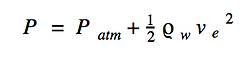top of page

### Thrust

Since the mass of the bottle system is changing, Newton’s Second Law cannot be directly applied.  This is because Newton’s Second Law relies on a body maintaining constant mass. Newton’s Third Law comes into play with the changing of mass.  Thrust is the movement or expulsion of mass from a body.  The general thrust equation is given below.It is known from Newton's Second Law that:The variable ve is the exhaust velocity of the ejected mass and (dM/dt) is the rate at which mass is ejected.  Now, with any system involving mass and velocity, energy or momentum equations should be used.  Starting with Bernoulli’s Energy equation for fluid flow and making a few assumptions, a new equation can be used.  Assume that the pressure difference due to the height of the water and that the velocity of the water on the ground compared to that of the water at the nozzle can be neglected.  With this assumption and the derivation shown above, it can be seen that:With this equation, a value for ve, or exit velocity can be found.  And this value can then be plugged into the thrust equation to get a more simplified equation.From this equation, it can be concluded that thrust is a function of pressure, which is a function of time, as well as the cross sectional area of the nozzle.

When graphing thrust versus time, it can be seen that thrust starts at a maximum and decreases over time.  Since the pressure in the bottle equilibrates with that of the pressure of the atmosphere, it is expected to decrease over time.bottom of page